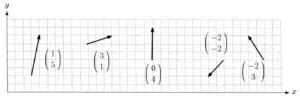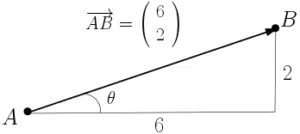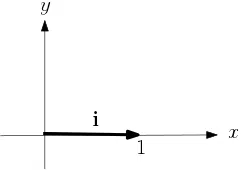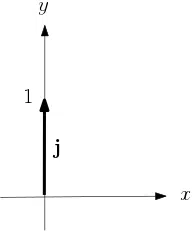# 2D VectorsVectors can be thought of as a way of storing multiple values. For column vectors, numbers are vertically stacked inside brackets. This is very much like when we use column vectors to describe translations at GCSE or similar. See Translation Vectors. Clearly, two-dimensional vectors have two entries – one for displacement in the $x$ direction and one for the $y$ direction. It follows that a vector can also be displayed as an arrow and can appear anywhere in the $x-y$ plane. A position vector, however, points specifically from the origin. See more on Position Vectors.

Bold or underlined letters are often used to identify vectors – it lets the reader know that more than one component is involved. Since two-dimensional vectors have components in two directions, they have a magnitude (size) and a direction described with an angle. It also means they can represent some quantities in mechanics – see Quantities & Units in Mechanics.

## Magnitude & Direction of 2D Vectors

In Mechanics, the magnitude of a velocity vector is speed. The magnitude of any vector is its length and can be found using Pythagoras.The notation $\overrightarrow{AB}$ is another way to express vectors. It identifies the vector that points from the point $A$ to the point $B$. The magnitude, which is the length of the vector, is then denoted $\vert\overrightarrow{AB}\vert$. Using Pythagoras,

$\vert\overrightarrow{AB}\vert=\sqrt{6^2+2^2}=\sqrt{40}=2\sqrt{10}$

See surds. The direction of the vector is the specific direction in which it points (and not how far) and can be described by an angle. In the diagram above, the angle $\theta$ is measured anti-clockwise relative to the horizontal (or $x$-axis) and is found using SOHCAHTOA: $\theta=\tan^{-1}\left(\frac{2}{6}\right)=18.4^\circ$ to 1 decimal place. The direction may also be indicated by an clockwise angle relative to the vertical (or $y$-axis), for example.  You need both magnitude and direction to fully describe a vector when not given it as a column vector.

Note that the vector $\overrightarrow{AB}$ points in the opposite direction to the vector $\overrightarrow{BA}$. In fact, $\overrightarrow{AB}=-\overrightarrow{BA}$. These vectors have the same magnitude but they differ in direction by $180^\circ$. See the examples below.

Also note that vectors are parallel if they are a multiple of one another (including negative numbers). For example, $\overrightarrow{AB}$, $3\overrightarrow{AB}$ and $\overrightarrow{BA}$ are all parallel. See Scalar Multiplication of Vectors.

## Unit VectorsUnit vectors are vectors that have a magnitude of 1. The standard unit vectors that point in the $x$ and $y$ directions respectively are given by ${\bf i}=\left(\begin{array}{c}1\\0\end{array}\right)$ and ${\bf j}=\left(\begin{array}{c}0\\1\end{array}\right)$.

Notice that $i$ and $j$ are given in bold to identify them as vectors. They may also be given as underlined letters.

It is possible to find a unit vector in any given direction. For example, the vector ${\bf a}=\left(\begin{array}{c}3\\4\end{array}\right)$ has magnitude $\vert{\bf a}\vert =5$. By dividing the entries by 5 (see Scalar Multiplication of Vectors), we obtain a unit vector in the direction of ${\bf a}$:

$\hat{{\bf a}} =\left(\begin{array}{c}3/5\\4/5\end{array}\right)$

where $\vert\hat{\bf a}\vert=1$. Note that a hat is often used to identify a unit vector. Hence why you may sometimes see the standard unit vectors with hats in place of dots.

## Examples

For the following vectors (also as seen above), find their magnitude, the anti-clockwise angle the vectors make with the horizontal and a unit vector that points in their given directions.Given that ${\bf a}=7{\bf i}-4{\bf j}$ and ${\bf b}=5{\bf j}-{\bf i}$ and the vector $2{\bf a}+\lambda {\bf b}$ is parallel to ${\bf j}$, find the value of $\lambda$.